# SCERT Maharashtra Question Bank solutions for 10th Standard SSC Mathematics 2 Geometry Maharashtra State Board 2021 chapter 3 - Circle [Latest edition]

#### Chapters## Chapter 3: Circle

Q.1Q.2Q.3Q.4Q.5Q.6Q.7Q.8
Q.1

### SCERT Maharashtra Question Bank solutions for 10th Standard SSC Mathematics 2 Geometry Maharashtra State Board 2021 Chapter 3 Circle Q.1

#### MCQ [1 Mark]

Q.1 | Q 1

Four alternative answers for the following question is given. Choose the correct alternative.

Two circles intersect each other such that each circle passes through the centre of the other. If the distance between their centres is 12, what is the radius of each circle?

• 6 cm

• 12 cm

• 24 cm

• can’t say

Q.1 | Q 2

Four alternative answers for the following question is given. Choose the correct alternative.

A circle touches all sides of a parallelogram. So the parallelogram must be a, ______

• rectangle

• rhombus

• square

• trapezium

Q.1 | Q 3

Four alternative answers for the following question is given. Choose the correct alternative.

∠ACB is inscribed in arc ACB of a circle with centre O. If ∠ACB = 65°, find m(arc ACB).

• 65°

• 130°

• 295°

• 230°

Q.1 | Q 4

Four alternative answers for the following question is given. Choose the correct alternative

In a cyclic ▢ABCD, twice the measure of ∠A is thrice the measure of ∠C. Find the measure of ∠C?

• 36

• 72

• 90

• 108

Q.1 | Q 5

Four alternative answers for the following question is given. Choose the correct alternative.

How many circles can drawn passing through three non-collinear points?

• 0

• Infinite

• 2

• One and only one (unique)

Q.1 | Q 6

Four alternative answers for the following question is given. Choose the correct alternative.

Two circles of radii 5.5 cm and 4.2 cm touch each other externally. Find the distance between their centres

• 9.7

• 1.3

• 2.6

• 4.6

Q.1 | Q 7

Four alternative answers for the following question is given. Choose the correct alternative.

What is the measurement of angle inscribed in a semicircle?

• 90°

• 120°

• 100°

• 60°

Q.1 | Q 8

Four alternative answers for the following question is given. Choose the correct alternative.

Two circles having diameters 8 cm and 6 cm touch each other internally. Find the distance between their centres.

• 2

• 14

• 7

• 1

Q.1 | Q 9

Four alternative answers for the following question is given. Choose the correct alternative.

Points A, B, C are on a circle, such that m(arc AB) = m(arc BC) = 120°. No point, except point B, is common to the arcs. Which is the type of ∆ABC?

• Equilateral triangle

• Scalene triangle

• Right angled triangle

• Isosceles triangle

Q.1 | Q 10

Four alternative answers for the following question is given. Choose the correct alternative.

In ▢PQRS if ∠RSP = 80° then find ∠RQT?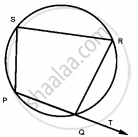• 100°

• 80°

• 70°

• 110°

Q.2

### SCERT Maharashtra Question Bank solutions for 10th Standard SSC Mathematics 2 Geometry Maharashtra State Board 2021 Chapter 3 Circle Q.2

#### Solve the following sub-questions [1 Mark]

Q.2 | Q 1

How many circles can be drawn passing through a point?

Q.2 | Q 2

Segment DP and segment DQ are tangent segments to the circle with center A. If DP = 7 cm. So find the length of the segment DQ.Q.2 | Q 3

Two circles having radii 3.5 cm and 4.8 cm touch each other internally. Find the distance between their centres.

Q.2 | Q 4

What is the measure of a semi circular arc?

Q.2 | Q 5

A, B, C are any points on the circle with centre O. If m(arc BC) = 110° and m(arc AB) = 125°, find measure arc AC.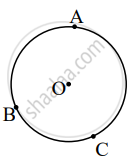Q.2 | Q 6

In the figure if ∠PQR = 50°, then find ∠PSR.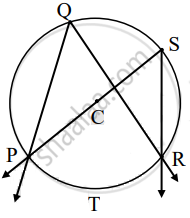Q.2 | Q 7

In the adjoining figure the radius of a circle with centre C is 6 cm, line AB is a tangent at A. What is the measure of ∠CAB? Why?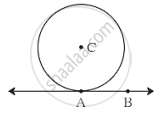Q.2 | Q 8

In the figure, quadrilateral ABCD is a cyclic. If ∠DAB = 75°, then find measure of ∠DCB.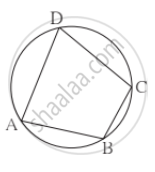Q.2 | Q 9

In the adjoining figure, seg DE is the chord of the circle with center C. seg CF⊥ seg DE and DE = 16 cm, then find the length of DF?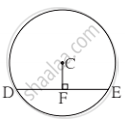Q.2 | Q 10

In the figure, if ∠ABC = 35°, then find m(arc AXC)?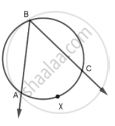Q.3

### SCERT Maharashtra Question Bank solutions for 10th Standard SSC Mathematics 2 Geometry Maharashtra State Board 2021 Chapter 3 Circle Q.3

#### Complete the following activities [2 Marks]

Q.3 | Q 1

The chords corresponding to congruent arcs of a circle are congruent. Prove the theorem by completing following activity.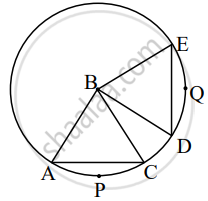Given: In a circle with centre B

arc APC ≅ arc DQE

To Prove: Chord AC ≅ chord DE

Proof: In ΔABC and ΔDBE,

side AB ≅ side DB    ......square

side BC ≅ side square    .....square

∠ABC ≅ ∠DBE    ......[Measure of congruent arcs]

∆ABC ≅ ∆DBE    ......square

Q.3 | Q 2

In figure, points G, D, E, F are concyclic points of a circle with centre C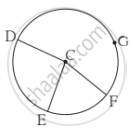∠ECF = 70°, m(arc DGF) = 200°

Find m(arc DEF) by completing activity.

m(arc EF) = ∠ECF     ......[Definition of measure of arc]

∴ m(arc EF) = square

But; m(arc DE)+ m(arc EF)+ m(arc DGF) = square   .....[Measure of a complete circle]

∴ m(arc DE) = square

∴ m(arc DEF) = m(arc DE) + m(arc EF)

∴ m(arc DEF) = square

Q.3 | Q 3

In the figure, if the chord PQ and chord RS intersect at point T, prove that: m∠STQ = 1/2 [m(arc PR) + m(arc SQ)] for any measure of ∠STQ by filling out the boxes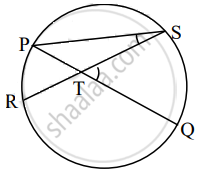Proof: m∠STQ = m∠SPQ + square  .....[Theorem of the external angle of a triangle]

= 1/2 m(arc SQ) + square   .....[Inscribed angle theorem]

= 1/2 [square + square]

Q.3 | Q 4

In figure, chord EF || chord GH. Prove that, chord EG ≅ chord FH. Fill in the blanks and write the proof.

Proof: Draw seg GF.∠EFG = ∠FGH     ......square    .....(I)

∠EFG = square   ......[inscribed angle theorem] (II)

∠FGH = square   ......[inscribed angle theorem] (III)

∴ m(arc EG) = square  ......[By (I), (II), व (III)]

chord EG ≅ chord FH   ........[corresponding chords of congruent arcs]

Q.3 | Q 5

The angle inscribed in the semicircle is a right angle. Prove the result by completing the following activity.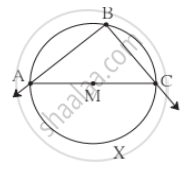Given: ∠ABC is inscribed angle in a semicircle with center M

To prove: ∠ABC is a right angle.

Proof: Segment AC is a diameter of the circle.

∴ m(arc AXC) = square

Arc AXC is intercepted by the inscribed angle ∠ABC

∠ABC = square      ......[Inscribed angle theorem]

= 1/2 xx square

∴ m∠ABC = square

∴ ∠ABC is a right angle.

Q.3 | Q 6

Prove that angles inscribed in the same arc are congruent.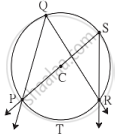Given: In a circle with center C, ∠PQR and ∠PSR are inscribed in same arc PQR. Arc PTR is intercepted by the angles.

To prove: ∠PQR ≅ ∠PSR.

Proof: m∠PQR = 1/2 xx ["m"("arc PTR"]   ......(i) square

m∠square = 1/2 xx ["m"("arc PTR"]   ......(ii) square

m∠square = m∠PSR      .....[By (i) and (ii)]

∴ ∠PQR ≅ ∠PSR

Q.3 | Q 7

If O is the center of the circle in the figure alongside, then complete the table from the given information.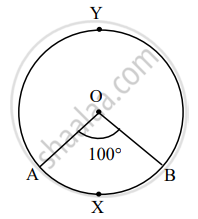The type of arc

 Type of circular arc Name of circular arc Measure of circular arc Minor arc Major arc
Q.4

### SCERT Maharashtra Question Bank solutions for 10th Standard SSC Mathematics 2 Geometry Maharashtra State Board 2021 Chapter 3 Circle Q.4

#### Solve the following sub-quesitons [2 Marks]

Q.4 | Q 1

In the adjoining figure, circle with centrer D touches the sides of ∠ACB at A and B. If ∠ACB = 52°, find measure of ∠ADB.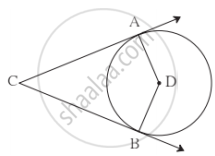Q.4 | Q 2

In the adjoining figure, the line MN touches the circle with center A at point M. If AN = 13 and MN = 5, then find the radius of the circle?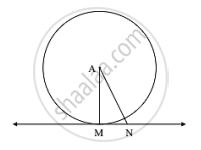Q.4 | Q 3

What is the distance between two parallel tangents of a circle having radius 4.5 cm ? Justify your answer.

Q.4 | Q 4

In figure, m(arc NS) = 125°, m(arc EF) = 37°, find the measure ∠NMS.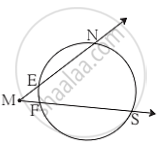Q.4 | Q 5

Length of a tangent segment drawn from a point which is at a distance 15 cm from the centre of a circle is 12 cm, find the diameter of the circle?

Q.4 | Q 6

In the figure, a circle with center C has m(arc AXB) = 100° then find central ∠ACB and measure m (arc AYB).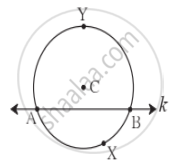Q.4 | Q 7. (1)

In figure, M is the centre of the circle and seg KL is a tangent segment. If MK = 12, KL = 6sqrt(3), then find Radius of the circle.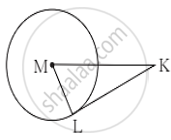Q.4 | Q 7. (2)

In figure, M is the centre of the circle and seg KL is a tangent segment. If MK = 12, KL = 6sqrt(3) , then find Measures of ∠K and ∠M.Q.4 | Q 8

In figure, chords AC and DE intersect at B. If ∠ABE = 108°, m(arc AE) = 95°, find m(arc DC).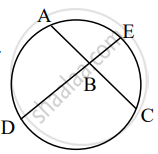Q.5

### SCERT Maharashtra Question Bank solutions for 10th Standard SSC Mathematics 2 Geometry Maharashtra State Board 2021 Chapter 3 Circle Q.5

#### Complete the following activity [3 Marks]

Q.5 | Q 1

Tangent segments drawn from an external point to a circle are congruent, prove this theorem. Complete the following activity.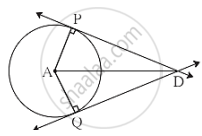Given: square

To Prove: square

Proof: Draw radius AP and radius AQ and complete the following proof of the theorem.

seg PA ≅ square      .....[Radii of the same circle]

seg AD ≅ seg AD    ......[square]

∠APD ≅ ∠AQD = 90°     .....[Tangent theorem]

∴ ∆PAD ≅ ∆QAD    ....[square]

∴ seg DP ≅ seg DQ  .....[square]

Q.5 | Q 2

MRPN is cyclic, ∠R = (5x – 13)°, ∠N = (4x + 4)°. Find measures of ∠R and ∠N, by completing the following activity.

Solution:  MRPN is cyclic

The apposite angles of a cyclic square are square

∠R + ∠N = square

∴ (5x – 13)° + (4x + 4) ° = square

∴ 9x = 189

∴ x = square

∴ ∠R = (5x – 13)° = square

∴ ∠N = (4x + 4)° = square

Q.5 | Q 3

In figure, seg AB is a diameter of a circle with centre O. The bisector of ∠ACB intersects the circle at point D. Prove that, seg AD ≅ seg BD. Complete the following proof by filling in the blanks.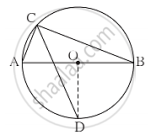Proof: Draw seg OD.

∠ACB = square     ......[Angle inscribed in semicircle]

∠DCB = square    ......[CD is the bisector of ∠C]

m(arc DB) = square   ......[Inscribed angle theorem]

∠DOB = square   ......[Definition of measure of an arc](i)

seg OA ≅ seg OB     ...... square (ii)

∴ Line OD is square of seg AB    ......[From (i) and (ii)]

∴ seg AD ≅ seg BD

Q.5 | Q 4

In the adjoining figure circles with centres X and Y touch each other at point Z. A secant passing through Z intersects the circles at points A and B respectively. Prove that, radius XA || radius YB.

Fill in the blanks and complete the proof.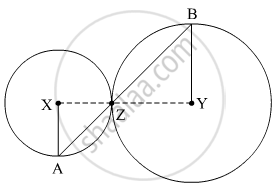Q.5 | Q 5

An exterior angle of a cyclic quadrilateral is congruent to the angle opposite to its adjacent interior angle, to prove the theorem complete the activity.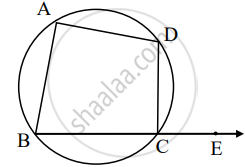Given:  ABCD is cyclic,

square is the exterior angle of  ABCD

Proof: square + ∠BCD = square    .....[Angles in linear pair] (I)

 ABCD is a cyclic.

square + ∠BAD = square     ......[Theorem of cyclic quadrilateral] (II)

By (I) and (II)

∠DCE + ∠BCD = square + ∠BAD

Q.5 | Q 6

Seg RM and seg RN are tangent segments of a circle with centre O. Prove that seg OR bisects ∠MRN as well as ∠MON with the help of activity.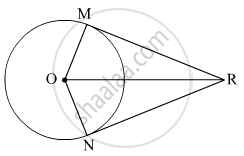Proof: In ∆RMO and ∆RNO,

∠RMO ≅ ∠RNO = 90°   ......[square]

hypt OR ≅ hypt OR    ......[square]

seg OM ≅ seg square    ......[Radii of the same circle]

∴ ∆RMO ≅ ∆RNO      ......[square]

∠MOR ≅ ∠NOR

Similairy ∠MRO ≅ square    ......[square]

Q.5 | Q 7

In the given figure, O is the centre of the circle. Seg AB, seg AC are tangent segments. Radius of the circle is r and l(AB) = r, Prove that, ▢ABOC is a square.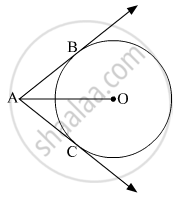Proof: Draw segment OB and OC.

l(AB) = r      ......[Given] (I)

AB = AC    ......[square] (II)

But OB = OC = r    ......[square] (III)

From (i), (ii) and (iii)

AB = square = OB = OC = r

∴ Quadrilateral ABOC is square

Similarly, ∠OBA = square      ......[Tangent Theorem]

If one angle of square is right angle, then it is a square.

∴ Quadrilateral ABOC is a square.

Q.6

### SCERT Maharashtra Question Bank solutions for 10th Standard SSC Mathematics 2 Geometry Maharashtra State Board 2021 Chapter 3 Circle Q.6

#### Solve the following sub-questions [3 Marks]

Q.6 | Q 1. i)

Prove the following theorems: Opposite angles of a cyclic quadrilateral are supplementry

Q.6 | Q 1. ii)

Prove the following theorems: Tangent segments drawn from an external point to the circle are congruent

Q.6 | Q 1. iii)

Prove the following theorems: Angles inscribed in the same arc are congruent

Q.6 | Q 2. (i)

Line ℓ touches a circle with centre O at point P. If radius of the circle is 9 cm, answer the following.

What is d(O, P) = ? Why?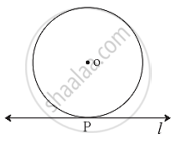Q.6 | Q 2. (ii)

Line ℓ touches a circle with centre O at point P. If radius of the circle is 9 cm, answer the following.

If d(O, Q) = 8 cm, where does the point Q lie?Q.6 | Q 2. (iiii)

Line ℓ touches a circle with centre O at point P. If radius of the circle is 9 cm, answer the following.

If d(O, R) = 15 cm, how many locations of point R are line on line l? At what distance will each of them be from point P?Q.6 | Q 3. (1)

In the adjoining figure, O is the centre of the circle. From point R, seg RM and seg RN are tangent segments touching the circle at M and N. If (OR) = 10 cm and radius of the circle = 5 cm, then What is the length of each tangent segment?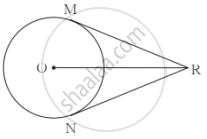Q.6 | Q 3. (2)

In the adjoining figure, O is the centre of the circle. From point R, seg RM and seg RN are tangent segments touching the circle at M and N. If (OR) = 10 cm and radius of the circle = 5 cm, then What is the measure of ∠MRO?Q.6 | Q 3. (3)

In the adjoining figure, O is the centre of the circle. From point R, seg RM and seg RN are tangent segments touching the circle at M and N. If (OR) = 10 cm and radius of the circle = 5 cm, then What is the measure of ∠MRN?Q.6 | Q 4

In the given figure, chord AB ≅ chord CD, Prove that, arc AC ≅ arc BD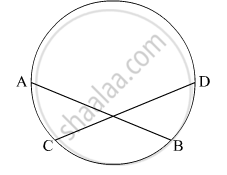Q.6 | Q 5. (1)

In figure, in a circle with centre O, length of chord AB is equal to the radius of the circle. Find measure of the following.

∠AOB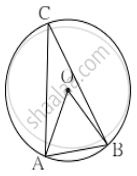Q.6 | Q 5. (2)

In figure, in a circle with centre O, length of chord AB is equal to the radius of the circle. Find measure of the following.

∠ACBQ.6 | Q 5. (3)

In figure, in a circle with centre O, length of chord AB is equal to the radius of the circle. Find measure of the following.

arc ABQ.6 | Q 6. (i)

In figure, chord LM ≅ chord LN, ∠L = 35°. Find m(arc MN)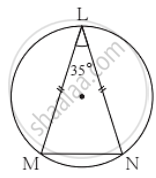Q.6 | Q 6. (ii)

In figure, chord LM ≅ chord LN, ∠L = 35°. Find m(arc LN)Q.6 | Q 7

Prove that, any rectangle is a cyclic quadrilateral

Q.6 | Q 8. (1)

In figure, PQRS is cyclic, side PQ ≅ side RQ, ∠PSR = 110°. Find measure of ∠PQR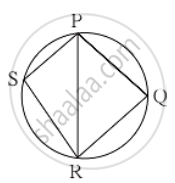Q.6 | Q 8. (2)

In figure, PQRS is cyclic, side PQ ≅ side RQ, ∠PSR = 110°. Find m(arc PQR)Q.6 | Q 8. (3)

In figure, PQRS is cyclic, side PQ ≅ side RQ, ∠PSR = 110°. Find m(arc QR)Q.6 | Q 9

In the figure, line l touches the circle with centre O at point P. Q is the midpoint of radius OP. RS is a chord through Q such that chords RS || line l. If RS = 12, find the radius of the circle.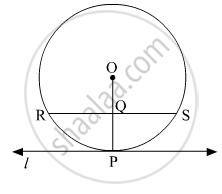Q.6 | Q 10. (1)

In figure, O is the centre of a circle, chord PQ ≅ chord RS If ∠POR = 70° and (arc RS) = 80°, find m(arc PR)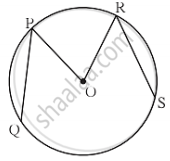Q.6 | Q 10. (2)

In figure, O is the centre of a circle, chord PQ ≅ chord RS If ∠POR = 70° and (arc RS) = 80°, find m(arc QS)Q.6 | Q 10. (3)

In figure, O is the centre of a circle, chord PQ ≅ chord RS If ∠POR = 70° and (arc RS) = 80°, find m(arc QSR)Q.6 | Q 11

In the adjoining figure circle with Centre Q touches the sides of ∠MPN at M and N. If ∠MPN = 40°, find measure of ∠MQN.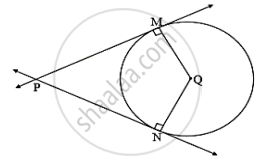Q.6 | Q 12

In the figure, if O is the center of the circle and two chords of the circle EF and GH are parallel to each other. Show that ∠EOG ≅ ∠FOH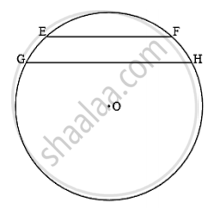Q.7

### SCERT Maharashtra Question Bank solutions for 10th Standard SSC Mathematics 2 Geometry Maharashtra State Board 2021 Chapter 3 Circle Q.7

#### Solve the following sub-questions [4 Marks]

Q.7 | Q 1

In the figure, segment PQ is the diameter of the circle with center O. The tangent to the tangent circle drawn from point C on it, intersects the tangents drawn from points P and Q at points A and B respectively, prove that ∠AOB = 90°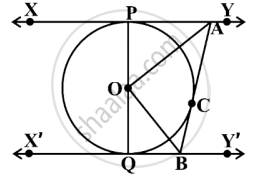Q.7 | Q 2

The chords AB and CD of the circle intersect at point M in the interior of the same circle then prove that CM × BD = BM × AC

Q.7 | Q 3

A circle with centre P is inscribed in the ∆ABC. Side AB, side BC and side AC touches the circle at points L, M and N respectively.
Radius of the circle is r.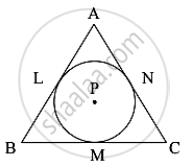Prove that : A(ΔABC) = 1/2 (AB + BC + AC) × r

Q.7 | Q 4

In the figure, ▢ABCD is a cyclic quadrilateral. If m(arc ABC) = 230°, then find ∠ABC , ∠CDA , ∠CBE.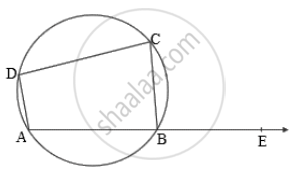Q.7 | Q 5

The figure ΔABC is an isosceles triangle with a perimeter of 44 cm. The sides AB and BC are congruent and the length of the base AC is 12 cm. If a circle touches all three sides as shown in the figure, then find the length of the tangent segment drawn to the circle from the point B.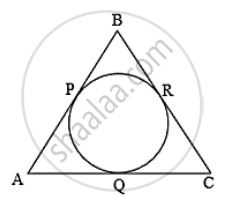Q.7 | Q 6

In the figure, ΔABC is an equilateral triangle. The angle bisector of ∠B will intersect the circumcircle ΔABC at point P. Then prove that: CQ = CA.Q.7 | Q 7

In the figure, quadrilateral ABCD is cyclic. If m(arc BC) = 90° and ∠DBC = 55°, then find the measure of ∠BCD.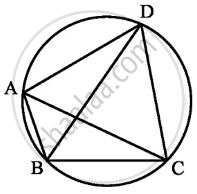Q.7 | Q 8

Given: A circle inscribed in a right angled ΔABC. If ∠ACB = 90° and the radius of the circle is r.

To prove: 2r = a + b – c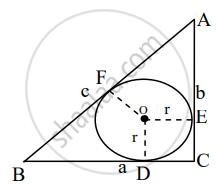Q.7 | Q 9

In a circle with centre P, chord AB is parallel to a tangent and intersects the radius drawn from the point of contact to its midpoint. If AB = 16sqrt(3), then find the radius of the circle

Q.7 | Q 10

In the figure, O is the center of the circle. Line AQ is a tangent. If OP = 3, m(arc PM) = 120°, then find the length of AP.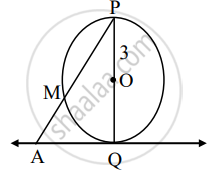Q.8

### SCERT Maharashtra Question Bank solutions for 10th Standard SSC Mathematics 2 Geometry Maharashtra State Board 2021 Chapter 3 Circle Q.8

#### Solve the following sub-questions [3 Marks]

Q.8 | Q 1

In the figure, O is the centre of the circle and ∠AOB = 90°, ∠ABC = 30°. Then find ∠CAB.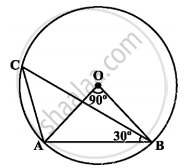Q.8 | Q 2

In the figure, a circle with center P touches the semicircle at points Q and C having center O. If diameter AB = 10, AC = 6, then find the radius x of the smaller circle.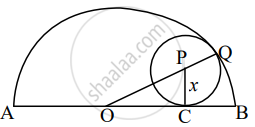Q.8 | Q 3

In the figure, a circle touches all the sides of quadrilateral ABCD from the inside. The center of the circle is O. If AD⊥ DC and BC = 38, QB = 27, DC = 25, then find the radius of the circle.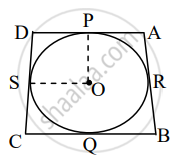Q.8 | Q 4

If AB and CD are the common tangents in the circles of two unequal (different) radii, then show that seg AB ≅ seg CD.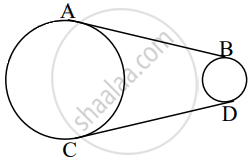Q.8 | Q 5

Circles with centres A, B and C touch each other externally. If AB = 36, BC = 32, CA = 30, then find the radii of each circle.

## Chapter 3: Circle

Q.1Q.2Q.3Q.4Q.5Q.6Q.7Q.8## SCERT Maharashtra Question Bank solutions for 10th Standard SSC Mathematics 2 Geometry Maharashtra State Board 2021 chapter 3 - Circle

SCERT Maharashtra Question Bank solutions for 10th Standard SSC Mathematics 2 Geometry Maharashtra State Board 2021 chapter 3 (Circle) include all questions with solution and detail explanation. This will clear students doubts about any question and improve application skills while preparing for board exams. The detailed, step-by-step solutions will help you understand the concepts better and clear your confusions, if any. Shaalaa.com has the Maharashtra State Board 10th Standard SSC Mathematics 2 Geometry Maharashtra State Board 2021 solutions in a manner that help students grasp basic concepts better and faster.

Further, we at Shaalaa.com provide such solutions so that students can prepare for written exams. SCERT Maharashtra Question Bank textbook solutions can be a core help for self-study and acts as a perfect self-help guidance for students.

Concepts covered in 10th Standard SSC Mathematics 2 Geometry Maharashtra State Board 2021 chapter 3 Circle are Theorem - Converse of Tangent at Any Point to the Circle is Perpendicular to the Radius, Number of Tangents from a Point on a Circle, Inscribed Angle Theorem, Intercepted Arc, Inscribed Angle, Property of Sum of Measures of Arcs, Tangent Segment Theorem, Converse of Tangent Theorem, Circles Passing Through One, Two, Three Points, Theorem of External Division of Chords, Theorem of Internal Division of Chords, Converse of Theorem of the Angle Between Tangent and Secant, Theorem of Angle Between Tangent and Secant, Converse: If a pair of opposite angles of a quadrilateral is supplementary, then the quadrilateral is cyclic., Corollary of Cyclic Quadrilateral Theorem, Theorem: Opposite angles of a cyclic quadrilateral are supplementary., Corollaries of Inscribed Angle Theorem, Angle Subtended by the Arc to the Point on the Circle, Angle Subtended by the Arc to the Centre, Introduction to an Arc, Touching Circles, Number of Tangents from a Point on a Circle, Tangent to a Circle, Tangents and Its Properties, Tangent Properties - If Two Circles Touch, the Point of Contact Lies on the Straight Line Joining Their Centers, Cyclic Properties, Tangent - Secant Theorem, Cyclic Quadrilateral, Concept of Circle - Centre, Radius, Diameter, Arc, Sector, Chord, Segment, Semicircle, Circumference, Interior and Exterior, Concentric Circles.

Using SCERT Maharashtra Question Bank 10th Standard [इयत्ता १० वी] solutions Circle exercise by students are an easy way to prepare for the exams, as they involve solutions arranged chapter-wise also page wise. The questions involved in SCERT Maharashtra Question Bank Solutions are important questions that can be asked in the final exam. Maximum students of Maharashtra State Board 10th Standard [इयत्ता १० वी] prefer SCERT Maharashtra Question Bank Textbook Solutions to score more in exam.

Get the free view of chapter 3 Circle 10th Standard [इयत्ता १० वी] extra questions for 10th Standard SSC Mathematics 2 Geometry Maharashtra State Board 2021 and can use Shaalaa.com to keep it handy for your exam preparation